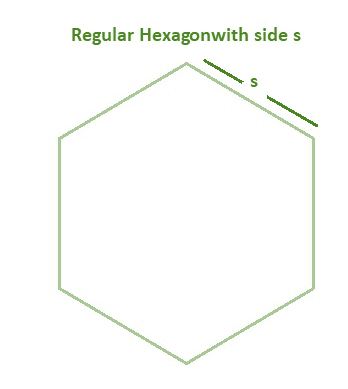GFG App
Open AppBrowser
Continue

# Regular Hexagon Formula

Hexagons are polygons that have six sides. Regular hexagons, irregular hexagons, and concave hexagons are the three different varieties of hexagons. The hexagon is called a Regular Hexagon if all of its sides are equal and all of its angles are the same. A regular pentagon has five equal sides, whereas a regular octagon has eight equal sides. When such prerequisites are not satisfied, polygons can take on the appearance of a variety of irregular shapes. When six equilateral triangles are placed side by side, a regular hexagon is formed. The area of the regular hexagon is thus six times the size of the identical triangle.Properties of Regular Hexagon

1. It has six equal sides and six identical angles.
2. It has six vertices.
3. The sum of the inner angles equals 720°.
4. The inside angle is 120° while the external angle is 60°.
5. It consists of six equilateral triangles.
6. Within a regular hexagon, 9 diagonals can be drawn.
7. All of the opposite sides are parallel.

### Regular Hexagon Formulae

There are specific formulae for the area of a regular hexagon and the perimeter of the regular hexagon. Let’s take a look at the regular hexagon formulae,

Area of Regular Hexagon

It is defined as the area occupied inside the boundary of a hexagon.

Area of an Hexagon = {(3√3a2 )/2}

Here ‘a’ represents the side of hexagon.

Perimeter of Regular Hexagon

The perimeter of a hexagon is defined as the length of the hexagon’s border. As a result, the perimeter will equal the total of the lengths of all sides. The perimeter of a hexagon may be calculated using the following formula,

Perimeter = 6 × side length of hexagon

P = 6s

### Sample Questions

Question 1: Calculate the area and perimeter of a regular hexagon whose side is 5 cm.

Solution:

Given, side of the hexagon = 5 cm

Area of an Hexagon = {(3√3a2 )/2}

= {[(3√3)52 ]/ 2}

= (3√3 × 25)/2

= 75√3 / 2

= (75 × 1.7320)/2

= 64.95 sq.cm

Perimeter of the hexagon = 6s

= 6 × 5

= 30 cm

So the area of hexagon is  64.95 sq.cm and perimeter of hexagon is 30 cm.

Question 2: Find the area of the board if the perimeter of a hexagonal board is 25 cm?

Solution:

Given, perimeter of the board = 25 cm

Perimeter of an Hexagon = 6s

25 = 6s

s = 25/6

= 4.166

Area of an Hexagon = {(3√3a2 )/2}

= {[3√3(4.166)2 ]/2}

= {[(3√3) 17.3555 ]/2}

= (3 × 1.7320 × 17.3555) /2

= 90.179 / 2

= 45.089 cm2.

Question 3:  A hexagonal board has a perimeter equal to 18 inches. Find its area.

Solution:

To Find: Area of the hexagon.

Given: Perimeter of hexagonal board = 18  inches.

The perimeter of hexagon = 6s

18 = 6s

s = 18/6

s = 3

Side = 3 inches.

Now Use the hexagon formula for Area,

Area of an Hexagon = {(3√3a2 )/2}

= {(3√3 × 32 )/2}

= {(3√3 × 9 ) /2}

= {3 × 1.7320 × 9} / 2

= 46.764 / 2

= 23.382 sq. inches

Question 4: Determine the side length of the regular hexagon whose perimeter is 42 units.

Solution:

To Find: Side length of hexagon

Given: Perimeter = 42 units.

Using the hexagon formula for perimeter

Perimeter of Regular hexagon = 6s

42 = 6s

Side = 42/6

Side = 7

So, the side length of the regular hexagon whose perimeter is 42 units is 7 unit.

Question 5: Evaluate the length of the side of a regular hexagon if its perimeter is given as 60 cm.

Solution:

Given, perimeter = 60 cm

Using the hexagon formula for perimeter

Perimeter of Regular hexagon = 6s

Therefore, 60 = 6s

Or, s = 60/6

= 10 cm
So, the length of the side of a regular hexagon whose perimeter 60 cm is 10 cm.

Question 6:  Find the area and perimeter of the hexagon, if all its sides have a length equal to 7 cm.

Solution:

To Find: Area of the hexagon and Perimeter of hexagon.

Given: Side = 7cm

The perimeter of hexagon = 6s

= 6 × 7

= 42 cm

Now Use the hexagon formula for Area,

Area of an Hexagon = {(3√3a2 )/2}

=  {(3√3 × 72 )/2}

= {(3√3 × 49 ) /2}

= {3 × 1.7320 × 49} / 2

= 254.60 / 2

= 127.302 sq. cm

My Personal Notes arrow_drop_up